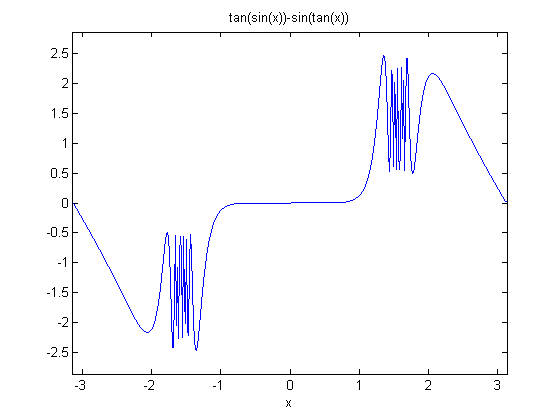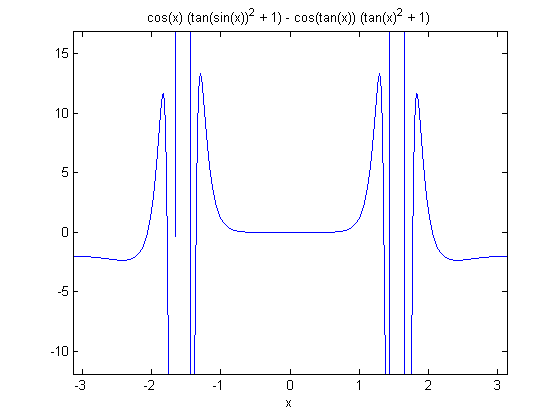# Supremum

Find the supremum of this function.

### Contents

#### Favorite Function

Here is one of my favorite functions. What is its maximum?

$$f(x) = \tan { \sin {x} } - \sin { \tan {x} }$$

Let's plot it with ezplot, which is pronounced easy-plot.

f = @(x) tan(sin(x)) - sin(tan(x))
ezplot(f,[-pi,pi])

f =

@(x)tan(sin(x))-sin(tan(x))The function is very flat at the origin. Its Taylor series begins with $x^7$. It oscillates infinitely often near $\pm \pi/2$. It is linear as it approaches zero again at $\pm \pi$. And, most important for our purposes here, ezplot has picked the limit on the y-axes to be between 2.5 and 3.

syms x
F = sym(f)
disp('taylor = ')
pretty(taylor(F,x,'order',10))
ylim = get(gca,'ylim')


F =

tan(sin(x)) - sin(tan(x))

taylor =

9    7
29 x    x
----- + --
756    30

ylim =

-2.867712755182179   2.867712755182179



#### Calculus

We learn in calculus that a maximum occurs at a zero of the derivative. But this function is not differentiable in the vicinity of $\pi/2$. The most interesting thing about an ezplot of the derivative is the title. Trying to find a zero of diff(F) is meaningless.

ezplot(diff(F),[-pi,pi])#### Sample

We can sample the function near $\pi/2$ to get a numerical approximation to the value of the maximum. Is that good enough?

x = 3*pi/8 + pi/4*rand(1,1000000);
y = f(x);
format long
smax = max(y)

smax =

2.557406355782225



#### Think

The computer has been a help, but we can do this without it.

$$\sin{x} \le 1$$

so

$$\sin{ \tan {x} } \le 1$$

and

$$\tan {\sin{x}} \le \tan {1}$$

Consequently

$$f(x) \le 1 + \tan {1}$$

#### Supremum

But I want to be a little more careful. As $x$ approaches $\pi/2$, $\tan{x}$ blows up. So $f(x)$ is actually not defined at $\pi/2$. For the domain of this function, one of the less than or equals changes to just a less than.

$$\sin{x} < 1$$

$$\tan {\sin{x}} < \tan {1}$$

$$f(x) < 1 + \tan {1}$$

The precise answer to my original question is that this function does not have a maximum. It has a "least upper bound" or supremum, the smallest quantity that the function does not exceed. The sup is:

$$\sup {f(x)} = 1 + \tan {1}$$

Now we can take a look at the numerical value.

sup = 1 + tan(1)

sup =

2.557407724654902



Published with MATLAB® 7.14

|